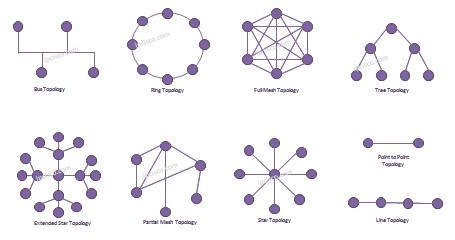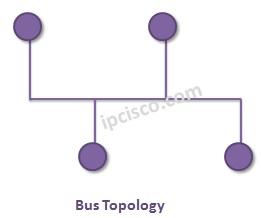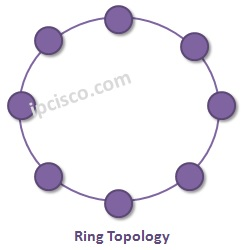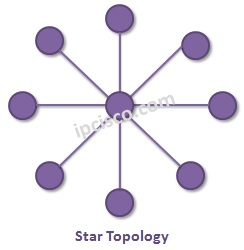# Network Topology Types# Network Topologies

Basically a network topology means that, connection style of the network nodes. To build a Network, we need a network topolog. There are different Network Topologies that can be used for different network scenarios. What are these Network Topologies?

These Network Topologies can be summarized like below :

• Bus
• Ring
• Star
• Extended Star
• Line
• Point-to-Point
• Partial Mesh
• Full Mesh
• TreeLet’s check these topology types one by one.

### Bus Topology

Bus Topology is the simplest LAN Network Topology. With Bus Topology, all the network nodes are connected to a common network media and only one node can receive and transmit data at a time.### Ring Topology

Ring Topology is a little complex topology. All the nodes are connected on a medium and frames are travels through one direction. If a node wants to send data, then it adds to this frame. Ring topology can be used as two different rings. With two ring, each ring can transmit data in different directions. This provides also a redundancy mechanism.### Star Topology

Star Topology is the topology that all the other nodes are connected to a central node. This is a widely used topology but it has a disadvantage that if the central device fails, the other nodes can not communicate.### Extended Star Topology

There is also an extended version of Star Topology called Extended Star. In this topology, there are end nodes and sub end nodes. All the sub end nodes are connected to end nodes. And they are conecteto the central node.Lesson tags: network topologies
Back to: HCNA > Network Basics